# Rheostat Range Limiting

## Chapter 3 - DC Circuits

PARTS AND MATERIALS

• Several 10 kΩ resistors
• One 10 kΩ potentiometer, linear taper (Radio Shack catalog # 271-1715)

CROSS-REFERENCES

Lessons In Electric Circuits, Volume 1, chapter 5: “Series and Parallel Circuits”

Lessons In Electric Circuits, Volume 1, chapter 7: “Series-Parallel Combination Circuits”

Lessons In Electric Circuits, Volume 1, chapter 8: “DC Metering Circuits”

LEARNING OBJECTIVES

• To determine series-parallel resistances
• To show calibration theory and practice

SCHEMATIC DIAGRAM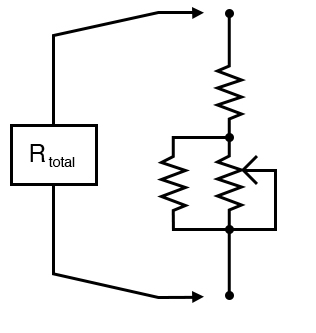ILLUSTRATION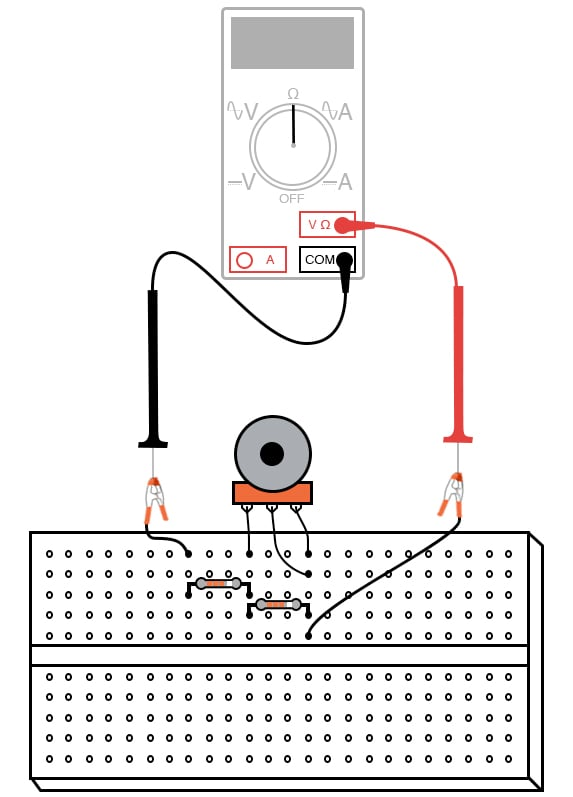INSTRUCTIONS

This experiment explores the different resistance ranges obtainable from combining fixed-value resistors with a potentiometer connected as a rheostat.

To begin, connect a 10 kΩ potentiometer as a rheostat with no other resistors connected.

Adjusting the potentiometer through its full range of travel should result in a resistance that varies smoothly from 0 Ω to 10,000 Ω: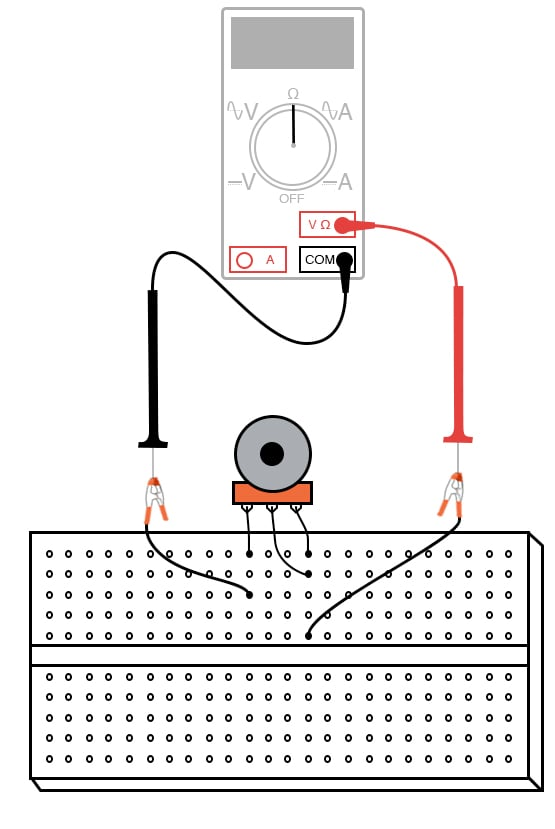Suppose we wanted to elevate the lower end of this resistance range so that we had an adjustable range from 10 kΩ to 20 kΩ with a full sweep of the potentiometer’s adjustment.

This could be easily accomplished by adding a 10 kΩ resistor in series with the potentiometer.

Add one to the circuit as shown and re-measure total resistance while adjusting the potentiometer: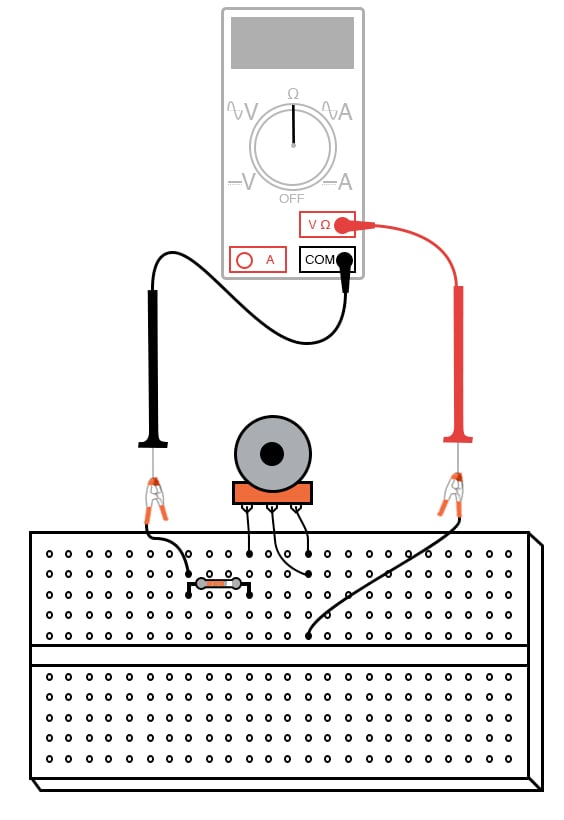A shift in the low end of an adjustment range is called a zero calibration, in metrological terms.

With the addition of a series 10 kΩ resistor, the “zero point” was shifted upward by 10,000 Ω.

The difference between high and low ends of a range—called the span of the circuit—has not changed, though: a range of 10 kΩ to 20 kΩ has the same 10,000 Ω span as a range of 0 Ω to 10 kΩ.

If we wish to shift the span of this rheostat circuit as well, we must change the range of the potentiometer itself.

We could replace the potentiometer with one of another value, or we could simulate a lower-value potentiometer by placing a resistor in parallel with it, diminishing its maximum obtainable resistance. This will decrease the span of the circuit from 10 kΩ to something less.

Add a 10 kΩ resistor in parallel with the potentiometer, to reduce the span to one-half of its former value: from 10 KΩ to 5 kΩ. Now the calibrated resistance range of this circuit will be 10 kΩ to 15 kΩ:There is nothing we can do to increase the span of this rheostat circuit, short of replacing the potentiometer with another of greater total resistance.

Adding resistors in parallel can only decrease the span. However, there is no such restriction with calibrating the zero point of this circuit, as it began at 0 Ω and may be made as great as we wish by adding resistance in series.

A multitude of resistance ranges may be obtained using only 10 KΩ fixed-value resistors, if we are creative with series-parallel combinations of them.

For instance, we can create a range of 7.5 kΩ to 10 kΩ by building the following circuit: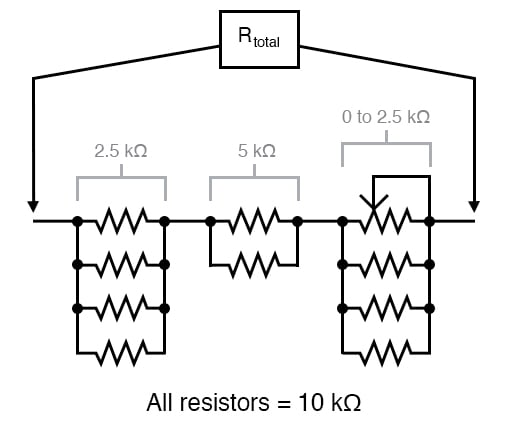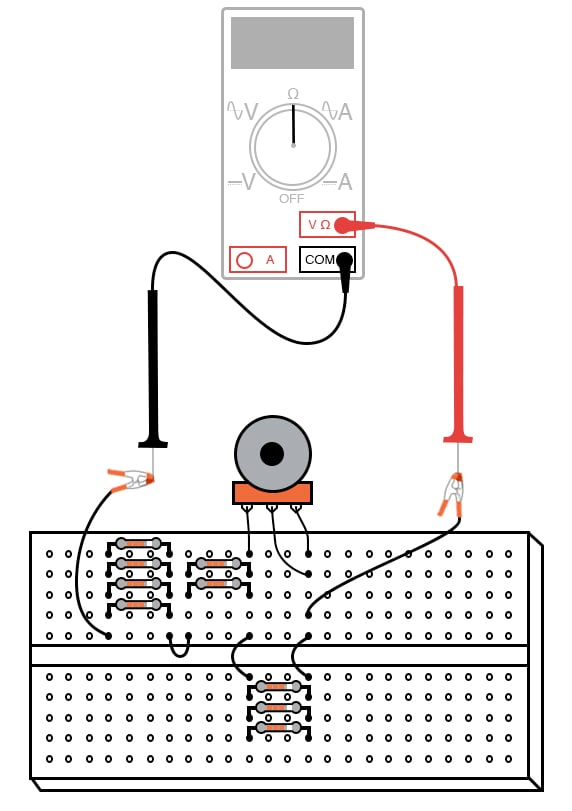Creating a custom resistance range from fixed-value resistors and a potentiometer is a very useful technique for producing precise resistances required for certain circuits, especially meter circuits.

In many electrical instruments—multimeters especially—resistance is the determining factor for the instrument’s range of measurement.

If an instrument’s internal resistance values are not precise, neither will its indications be.

Finding a fixed-value resistor of just the right resistance for placement in an instrument circuit design is unlikely, so custom resistance “networks” may need to be built to provide the desired resistance.

Having a potentiometer as part of the resistor network provides a means of correction if the network’s resistance should “drift” from its original value.

Designing the network for minimum span ensures that the potentiometer’s effect will be small, so that precise adjustment is possible and so that accidental movement of its mechanism will not result in severe calibration errors.

Experiment with different resistor “networks” and note the effects on total resistance range.

RELATED WORKSHEETS: